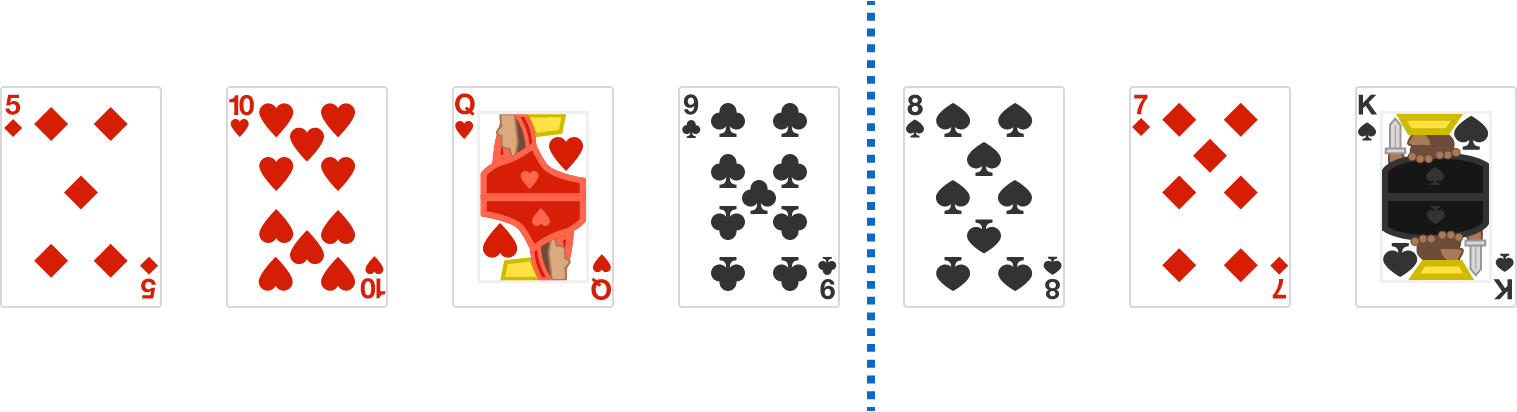# Splitting Piles

Probability Level 1

You are given a set of cards arranged in a line. Each card is either $\color{#333333}{\text{black}}$ or $\color{#D61F06}{\text{red}}$. You may divide the cards into two parts by picking a line before or after the whole cards (so that a part can contain 0 cards), or somewhere between two cards.

No matter how many cards are arranged in whatever manner, can you always ensure the number of $\color{#333333}{\text{black}}$ cards in the left part is exactly the same as the number of $\color{#D61F06}{\text{red}}$ cards in the right part?

An example split that fulfills the condition is shown below.There is 1 $\color{#333333}{\text{black}}$ card in the left part and 1 $\color{#D61F06}{\text{red}}$ card in the right part

×